Test: Power Energy

# Test: Power Energy

Test Description

## 20 Questions MCQ Test Basic Electrical Technology | Test: Power Energy

Test: Power Energy for Electrical Engineering (EE) 2022 is part of Basic Electrical Technology preparation. The Test: Power Energy questions and answers have been prepared according to the Electrical Engineering (EE) exam syllabus.The Test: Power Energy MCQs are made for Electrical Engineering (EE) 2022 Exam. Find important definitions, questions, notes, meanings, examples, exercises, MCQs and online tests for Test: Power Energy below.
Solutions of Test: Power Energy questions in English are available as part of our Basic Electrical Technology for Electrical Engineering (EE) & Test: Power Energy solutions in Hindi for Basic Electrical Technology course. Download more important topics, notes, lectures and mock test series for Electrical Engineering (EE) Exam by signing up for free. Attempt Test: Power Energy | 20 questions in 20 minutes | Mock test for Electrical Engineering (EE) preparation | Free important questions MCQ to study Basic Electrical Technology for Electrical Engineering (EE) Exam | Download free PDF with solutions
 1 Crore+ students have signed up on EduRev. Have you?
Test: Power Energy - Question 1

### Which of the following is not an expression power?

Detailed Solution for Test: Power Energy - Question 1

Power is the product of voltage and current. Writing I in terms of V, we get P=V2/R and writing V in terms of I, we get P=I2r.

Test: Power Energy - Question 2

### Which of the following statements are true?

Detailed Solution for Test: Power Energy - Question 2

Power is proportional to both voltage and current. Hence both the options are right.

Test: Power Energy - Question 3

### A 250V bulb passes a current of 0.3A. Calculate the power in the lamp.

Detailed Solution for Test: Power Energy - Question 3

Here, V = 250v and I = 0.3A. P=VI. Which implies that, P=250*0.3=75W.

Test: Power Energy - Question 4

Kilowatt-hour(kWh) is a unit of?

Detailed Solution for Test: Power Energy - Question 4

Power is the energy per unit time. That is, P=E/t. If the unit of power is kW and the unit of time is hour, then the unit of energy=unit of power*unit of time=kWh.

Test: Power Energy - Question 5

Calculate the power in the 20 ohm resistance.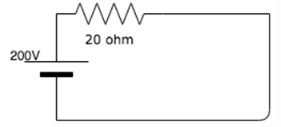Detailed Solution for Test: Power Energy - Question 5

Here V = 200v and Resistance( R) = 20ohm. P=V2/R= 2002/20=2000W=2kW

Test: Power Energy - Question 6

A current of 5A flows in a resistor of 2 ohm. Calculate the power in the resistor and the energy dissipated in 300 seconds.

Detailed Solution for Test: Power Energy - Question 6

P = I2R = 52*2 = 50W.
E = Pt = 50*300 = 15000J = 15kJ.

Test: Power Energy - Question 7

Calculate the power across each 20 ohm resistance.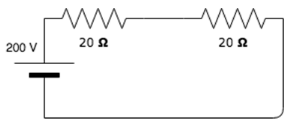Detailed Solution for Test: Power Energy - Question 7

This is a series connected circuit hence the current across each resistance is the same. To find current: I=V/R=200/40=5A.
To find power: P=I2R=(5)2*20 = 500W. Since both the resistors have a resistance of 20 ohm, the power across both is the same.

Test: Power Energy - Question 8

Calculate the power across each 10 ohm resistance.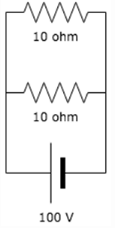Detailed Solution for Test: Power Energy - Question 8

This parallel connected circuit, hence the voltage across each of the resistors are the same. P=V2/R= 1002/10=1000W=1kW. Since both the resistors receive the same amount of voltage, the Power in both are the same.

Test: Power Energy - Question 9

Calculate the work done in a resistor of 20 ohm carrying 5A of current in 3 hours.

Detailed Solution for Test: Power Energy - Question 9

To find power: P=I2R=52*20=500W=0.5kW.
To find Work done: W=Pt=0.5*3=1.5kWh.

Test: Power Energy - Question 10

The SI unit of power is?

Detailed Solution for Test: Power Energy - Question 10

Power = energy/time =J/s(joules per second). Hence the Si unit of Power is J/s.

Test: Power Energy - Question 11

Which among the following is a unit for electrical energy?

Detailed Solution for Test: Power Energy - Question 11

Watt is a unit of power and hour is a unit of time. Energy is the product of power and time, hence the unit for power is kWh.

Test: Power Energy - Question 12

A bulb has a power of 200W. What is the energy dissipated by it in 5 minutes?

Detailed Solution for Test: Power Energy - Question 12

Here, Power = 200w and time = 5min. E=Pt => E= 200*5= 1000Wmin=60000Ws= 60000J= 60kJ.

Test: Power Energy - Question 13

Out of the following, which one is not a source of electrical energy?

Detailed Solution for Test: Power Energy - Question 13

A potentiometer is an instrument used for measuring voltage hence it is not a source for electrical energy.

Test: Power Energy - Question 14

Calculate the energy dissipated by the circuit in 50 seconds.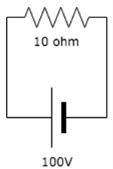Detailed Solution for Test: Power Energy - Question 14

Here V = 100 and R = 10. Power in the circuit= V2/R= 1002/10= 1000W.
Energy= Pt= 1000*50= 50000J= 50kJ.

Test: Power Energy - Question 15

Which among the following is an expression for energy?

Detailed Solution for Test: Power Energy - Question 15

Expression for power= VI, substituting I from ohm’s law we can write, P=V2/R. Energy is the product of power and time, hence E=Pt= V2t/R.

Test: Power Energy - Question 16

Calculate the energy in the 10 ohm resistance in 10 seconds.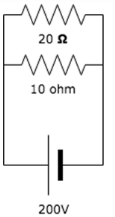Detailed Solution for Test: Power Energy - Question 16

Since the resistors are connected in parallel, the voltage across both the resistors are the same, hence we can use the expression P=V2/R. P=2002/10= 4000W. E=Pt= 4000*10=40000Ws= 40000J= 40kJ.

Test: Power Energy - Question 17

A battery converts___________

Detailed Solution for Test: Power Energy - Question 17

A battery is a device in which the chemical elements within the battery react with each other to produce electrical energy.

Test: Power Energy - Question 18

A current of 2A flows in a wire offering a resistance of 10ohm. Calculate the energy dissipated by the wire in 0.5 hours.

Detailed Solution for Test: Power Energy - Question 18

Here I (current) = 2A and Resistance(R) = 10ohm. Power= I2R= 22*10=40. Energy=Pt= 40*0.5*60*60= 72000J=72kJ.

Test: Power Energy - Question 19

Calculate the energy in the 5 ohm resistor in 20 seconds.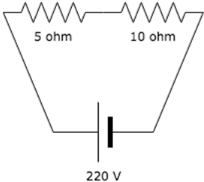Detailed Solution for Test: Power Energy - Question 19

The current in the circuit is equal to the current in the 5 ohm resistor since it a series connected circuit, hence I=220/(5+10)=14.67A. P=I2R= 14.672*5=1075.8W. E=Pt= 1075.8*20= 21516J=21.5kJ.

Test: Power Energy - Question 20

Practically, if 10kJ of energy is supplied to a device, how much energy will the device give back?

Detailed Solution for Test: Power Energy - Question 20

Practically, if 10kJ of energy is supplied to a system, it returns less than the supplied energy because, some of the energy is lost as heat energy, sound energy etc.

## Basic Electrical Technology

57 docs|59 tests
 Use Code STAYHOME200 and get INR 200 additional OFF Use Coupon Code
Information about Test: Power Energy Page
In this test you can find the Exam questions for Test: Power Energy solved & explained in the simplest way possible. Besides giving Questions and answers for Test: Power Energy, EduRev gives you an ample number of Online tests for practice

57 docs|59 tests